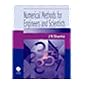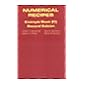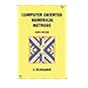Normal view MARC view ISBD view

# Numerical recipes example book (C) / William T. Vetterling ... [et al.].

Material type:TextPublisher: Cambridge ; New York : Cambridge University Press, 1992Edition: 2nd ed.Description: viii, 325 p. ; 24 cm.ISBN: 0521437202; 9780521437202.DDC classification: 519.4028 Online resources: Publisher description | WorldCat details | E-Book Fulltext
Contents:
Table of contents Preface; 1. Preliminaries; 2. Solution of linear algebraic equations; 3. Interpolation and extrapolation; 4. Integration of functions; 5. Evaluation of functions; 6. Special functions; 7. Random numbers; 8. Sorting; 9. Root finding and nonlinear sets of equations; 10. Minimization and maximization of functions; 11. Eigensystems; 12. Fast Fourier transform; 13. Fourier and spectral applications; 14. Statistical description of data; 15. Modeling of data; 16. Integration of ordinary differential equations; 17. Two point boundary value problems; 18. Integral equations and inverse theory; 19. Partial differential equations; 20. Less-numerical algorithms; Index of demonstrated subroutines.
Summary: The example books published as part of the Numerical Recipes second edition series contain source programs that exercise and demonstrate all of the Numerical Recipes subroutines.
Tags from this library: No tags from this library for this title.Average rating: 0.0 (0 votes)
Item type Current location Collection Call number Copy number Status Date due Barcode Item holdsE-Book
E-book
Non-fiction 519.4028 NUM 1992 (Browse shelf) Not for loanText
Reserve Section
Non-fiction 519.4028 NUM 1992 (Browse shelf) C-1 Not For Loan 7163Text
Circulation Section
Non-fiction 519.4028 NUM 1992 (Browse shelf) C-2 Available 7164
Total holds: 0
##### Browsing EWU Library Shelves , Shelving location: Reserve Section Close shelf browser
 No cover image available519.4 SCN 1996 Numerical mathematical analysis / 519.4 SHN 2004 Numerical methods for engineers and scientists / 519.4 SHN 2004 Numerical methods for engineers and scientists / 519.4028 NUM 1992 Numerical recipes example book (C) / 519.4'0285 RAC 1993 Computer oriented numerical methods / 519.4'0285 RAC 1993 Computer oriented numerical methods / 519.40285 RAC 1983 Computer oriented numerical methods /

Includes index.

Table of contents Preface; 1. Preliminaries; 2. Solution of linear algebraic equations; 3. Interpolation and extrapolation; 4. Integration of functions; 5. Evaluation of functions; 6. Special functions; 7. Random numbers; 8. Sorting; 9. Root finding and nonlinear sets of equations; 10. Minimization and maximization of functions; 11. Eigensystems; 12. Fast Fourier transform; 13. Fourier and spectral applications; 14. Statistical description of data; 15. Modeling of data; 16. Integration of ordinary differential equations; 17. Two point boundary value problems; 18. Integral equations and inverse theory; 19. Partial differential equations; 20. Less-numerical algorithms; Index of demonstrated subroutines.

The example books published as part of the Numerical Recipes second edition series contain source programs that exercise and demonstrate all of the Numerical Recipes subroutines.

Electronics & Communications Engineering Electrical & Electronic Engineering

There are no comments for this item.